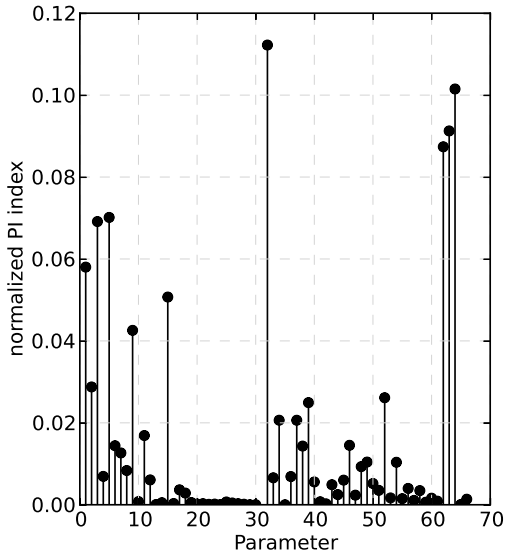## REKLIM

### Sensitivity Analysis of a complex Land Surface ModelLand surface models require a large number of parameters. These parameters are hard-coded as constants in the models despite they are obtained e.g. via regression methods from field data and have therefore an uncertainty range.

In this projcet we study the effect of parametric sensitivity of the model output. We apply a global sensitivity design using stratified random sampled parameter values and conducting local derivative based parameter pertubations. The relative sensitivity of three model outputs, latent and sensible heat as well as photosynthesis, is captured via an objective function of normalized squared flux deviations. For determine sensitive model parameters and their mean relative effect on the model outputs, we apply an eigendecomposition on a matrix containing numerical information of the objective function. After introducing a parameter importance index, see Figure, we propose a new criterion acting on this index, to determine the important model parameters depenent on a user specific confidence level for e.g. parameter estimation purposes.

We use the Community Land Model (CLM, rev3.5) from the National Center of Atmospheric Research, Boulder, CO (http://www.cgd.ucar.edu/tss/clm/).

Contact: ,